Next: The Sierpinski gasket Up: A turtle in a Previous: Fractal Dimension

# Cantor sets

The set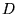of the previous section is an example of a Cantor set. Often, a Cantor set is described as the result of an iterative process, much in the same way we described the Koch curve in §2. We'll describe the most common Cantor set, the middle-thirds Cantor set'', this way now.

Begin with the interval [0, 1] as the set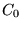. Now remove the open interval (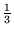,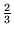) fromto obtain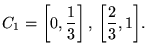To get the second stage, we remove the middle third of each interval in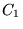. This gives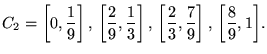We continue in this way, removing the middle third of each interval into obtain(See Fig. 3). Note that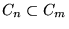for all n > m. What is left as we let n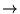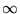is the Cantor set. That is,It may not be clear at first that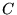even exists. It seems we are taking just about everything out when we remove the intervals. Indeed, the total length of intervals we remove is++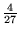+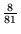+...= 1.

That is, the total length of the segments removed is equal to the segment we started with! For this reason, a set likeis sometimes called a Cantor dust''. But notice that the endpoints of each interval ofmust be in, because they never get removed at any level. Thus,is nonempty, since it contains at least the points0, 1,,,,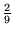,,,,,.... And it contains quite a few more points, as well. To see that, we can viewin a manner similar to the description of the setgiven in §5.2. However,is more naturally described in base 3, rather than base 10. Let's briefly review what we mean by this.

When we express a number x in base 10, we are expressing it as sums of powers of ten. Thus, when we write e5148.413, we are actually saying that

e5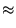1 . 102 + 4 . 101 + 8 . 100 + 4 . 10-1 + 1 . 10-2 + 3 . 10-3.

There is nothing magic about powers of 10, of course (except that it is the base we learned as children, and the base used by just about every living human5.14for everyday numbers). For example, we could express this5.15in ternary (that is, base 3), where we would have

e512111.1020113 = 1 . 34 + 2 . 33 + 1 . 32 + 1 . 31 + 1 . 30 + 1 . 3-1 + 0 . 3-2 + 2 . 3-3 + 0 . 3-4 + 1 . 3-5 + 1 . 3-6.

In computer applications, the bases 16 (hexadecimal) and 2 (binary) are very common, because the computer represents everything internally in binary.5.16

Returning to the Cantor set, note that when we remove the interval (1/3, 2/3), we are removing all the numbers whose ternary expansion has a 1 immediately after the decimal place.5.17At the next stage, we remove those points which have a 1 in the second place after the decimal, and so on. Thus, in the limit, we obtain the following alternate description of: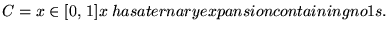You may remember that rational numbers correspond to numbers with a decimal expansion that eventually repeats; the same holds true of the expansion in any base (how might you prove this if you assume it for decimals?). It is easy to see using this description thatis closed (that is, it contains all its limit points), totally disconnected, and contains an uncountable number of elements (there are a lot of sequences of 0s and 2s), just like the setof the previous section. It is also self-similar, because if we take any small section of it and expand it by an appropriate power of 3, it looks like the entire set.

What is its box dimension? The description by the setsgive us an effective way to calculate the limit: at the nth stage, we have 2n intervals of length 3-n. Using this, we can immediately calculate that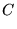=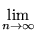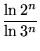=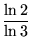0.63093.

You may have noticed the strong similarity in the construction of the Koch curve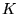of §2 and the middle-thirds Cantor set. In fact, notice that the intersection ofwith its base (the initial segment) is exactly the middle-thirds Cantor set.

#### Footnotes

... human5.14
Some Native American peoples used base 20, and the Babylonians were very fond of base 12 and base 60 (consider how we measure time).
... this5.15
Maple will convert integers to other bases, using a command such as convert(148, base, 3). One has to work a little bit to convert fractions-- can you think of a way to do this?
... binary.5.16
Hexadecimal is convenient for use with a binary system, because we can group blocks of 4 binary digits to obtain one hexadecimal digit (by convention, the letters A-F are used to represent the integers 10-15). This is quite handy in computer applications, because a single byte is represented by 8 binary digits (bits), or two hex digits. Before 8-bit bytes became standard, octal (base 8) was very common as well. Octal is much less convenient for 8-bit quantities, however, because 8 bits don't break up nicely into groups of 3 bits.
... place.5.17
As in decimal expansions, some points have two representations. Thus, the point 1/3 can be written either as .13 or as .0222.... As in the construction ofin §5.2, we keep such points in our set.Next: The Sierpinski gasket Up: A turtle in a Previous: Fractal Dimension

Translated from LaTeX by Scott Sutherland
2002-08-29